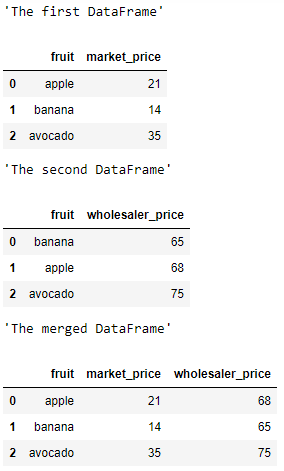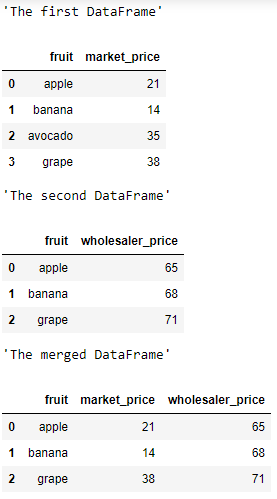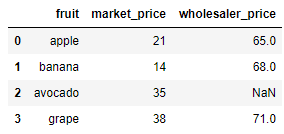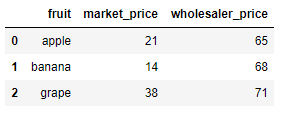Open in App
Not now

# Joining two Pandas DataFrames using merge()

• Difficulty Level : Medium
• Last Updated : 17 Aug, 2020

Let us see how to join two Pandas DataFrames using the `merge()` function.

## merge()

Syntax : DataFrame.merge(parameters)

Parameters :

• right : DataFrame or named Series
• how : {‘left’, ‘right’, ‘outer’, ‘inner’}, default ‘inner’
• on : label or list
• left_on : label or list, or array-like
• right_on : label or list, or array-like
• left_index : bool, default False
• right_index : bool, default False
• sort : bool, default False
• suffixes : tuple of (str, str), default (‘_x’, ‘_y’)
• copy : bool, default True
• indicator : bool or str, default False
• validate : str, optional

Returns : A DataFrame of the two merged objects.

Example 1 : Merging two Dataframe with same number of elements :

 `# importing the module``import` `pandas as pd`` ` `# creating the first DataFrame``df1 ``=` `pd.DataFrame({``"fruit"` `: [``"apple"``, ``"banana"``, ``"avocado"``],``                    ``"market_price"` `: [``21``, ``14``, ``35``]})``display(``"The first DataFrame"``)``display(df1)`` ` `# creating the second DataFrame``df2 ``=` `pd.DataFrame({``"fruit"` `: [``"banana"``, ``"apple"``, ``"avocado"``],``                    ``"wholesaler_price"` `: [``65``, ``68``, ``75``]})``display(``"The second DataFrame"``)``display(df2)`` ` `# joining the DataFrames``display(``"The merged DataFrame"``)``pd.merge(df1, df2, on ``=` `"fruit"``, how ``=` `"inner"``)`

Output :Example 2 : Merging two Dataframe with different number of elements :

 `# importing the module``import` `pandas as pd`` ` `# creating the first DataFrame``df1 ``=` `pd.DataFrame({``"fruit"` `: [``"apple"``, ``"banana"``, ``                               ``"avocado"``, ``"grape"``],``                    ``"market_price"` `: [``21``, ``14``, ``35``, ``38``]})``display(``"The first DataFrame"``)``display(df1)`` ` `# creating the second DataFrame``df2 ``=` `pd.DataFrame({``"fruit"` `: [``"apple"``, ``"banana"``, ``"grape"``],``                    ``"wholesaler_price"` `: [``65``, ``68``, ``71``]})``display(``"The second DataFrame"``)``display(df2)`` ` `# joining the DataFrames``# here both common DataFrame elements are in df1 and df2, ``# so it extracts apple, banana, grapes from df1 and df2.  ``display(``"The merged DataFrame"``)``pd.merge(df1, df2, on ``=` `"fruit"``, how ``=` `"inner"``)`

Output :If we use `how = "Outer"`, it returns all elements in df1 and df2 but if element column are null then its return NaN value.

 `pd.merge(df1, df2, on ``=` `"fruit"``, how ``=` `"outer"``)`

Output :If we use `how = "left"`, it returns all the elements that present in the left DataFrame.

 `pd.merge(df1, df2, on ``=` `"fruit"``, how ``=` `"left"``)`

Output :If we use `how = "right"`, it returns all the elements that present in the right DataFrame.

 `pd.merge(df1, df2, on ``=` `"fruit"``, how ``=` `"right"``)`

Output :My Personal Notes arrow_drop_up